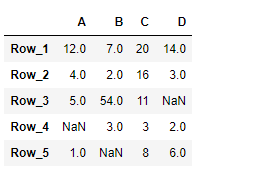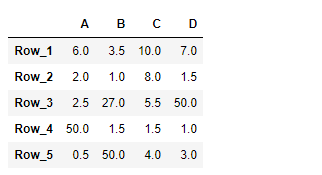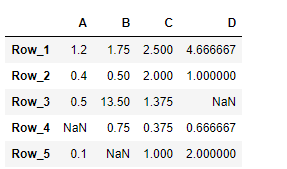Open in App
Not now

# Python | Pandas DataFrame.truediv

• Last Updated : 21 Feb, 2019

Pandas DataFrame is a two-dimensional size-mutable, potentially heterogeneous tabular data structure with labeled axes (rows and columns). Arithmetic operations align on both row and column labels. It can be thought of as a dict-like container for Series objects. This is the primary data structure of the Pandas.

Pandas` DataFrame.truediv()` function perform the floating division of dataframe and other, element-wise. It is equivalent to `dataframe / other`, but with support to substitute a fill_value for missing data in one of the inputs.

Syntax: DataFrame.truediv(other, axis=’columns’, level=None, fill_value=None)

Parameter :
other : scalar, sequence, Series, or DataFrame
axis : {0 or ‘index’, 1 or ‘columns’}
level : Broadcast across a level, matching Index values on the passed MultiIndex level.
fill_value : Fill existing missing (NaN) values, and any new element needed for successful DataFrame alignment.

Returns : Result of the arithmetic operation.

Example #1 : Use `DataFrame.truediv()` function to perform division of the given dataframe with a scalar element-wise. Also fill 100 at the place of all the missing values.

 `# importing pandas as pd ` `import` `pandas as pd ` ` `  `# Creating the DataFrame ` `df ``=` `pd.DataFrame({``"A"``:[``12``, ``4``, ``5``, ``None``, ``1``],  ` `                   ``"B"``:[``7``, ``2``, ``54``, ``3``, ``None``],  ` `                   ``"C"``:[``20``, ``16``, ``11``, ``3``, ``8``],  ` `                   ``"D"``:[``14``, ``3``, ``None``, ``2``, ``6``]})  ` ` `  `# Create the index ` `index_ ``=` `[``'Row_1'``, ``'Row_2'``, ``'Row_3'``, ``'Row_4'``, ``'Row_5'``] ` ` `  `# Set the index ` `df.index ``=` `index_ ` ` `  `# Print the DataFrame ` `print``(df) `

Output :Now we will use `DataFrame.truediv()` function to perform division of the given dataframe by 2, element-wise. We are going to fill 100 at the place of all the missing values in this dataframe.

 `# divide by 2 element-wise ` `# fill 100 at the place of missing values ` `result ``=` `df.truediv(other ``=` `2``, fill_value ``=` `100``) ` ` `  `# Print the result ` `print``(result) `

Output :As we can see in the output, the `DataFrame.truediv()` function has successfully performed the division of the given dataframe by a scalar.

Example #2 : Use `DataFrame.truediv()` function to perform the division of the given dataframe using a list.

 `# importing pandas as pd ` `import` `pandas as pd ` ` `  `# Creating the DataFrame ` `df ``=` `pd.DataFrame({``"A"``:[``12``, ``4``, ``5``, ``None``, ``1``],  ` `                   ``"B"``:[``7``, ``2``, ``54``, ``3``, ``None``],  ` `                   ``"C"``:[``20``, ``16``, ``11``, ``3``, ``8``],  ` `                   ``"D"``:[``14``, ``3``, ``None``, ``2``, ``6``]})  ` ` `  `# Create the index ` `index_ ``=` `[``'Row_1'``, ``'Row_2'``, ``'Row_3'``, ``'Row_4'``, ``'Row_5'``] ` ` `  `# Set the index ` `df.index ``=` `index_ ` ` `  `# Print the DataFrame ` `print``(df) `

Output :Now we will use `DataFrame.truediv()` function to perform division of the given dataframe using a list.

 `# divide using a list ` `# across the column axis ` `result ``=` `df.truediv(other ``=` `[``10``, ``4``, ``8``, ``3``], axis ``=` `1``) ` ` `  `# Print the result ` `print``(result) `

Output :As we can see in the output, the `DataFrame.truediv()` function has successfully performed the division of the given dataframe by a list.

My Personal Notes arrow_drop_up
Related Articles